User:Jouvencel

Control command based of quaternions

Files :

- stabilization_attitude_quat_int.c (.h)

- stabilization_attitude_ref_quat_int.c (.h)

- quat_setpoint_int.c (.h)

• Position in the autopilot structure
• Control structure

note : (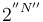$2^{''N''}$) number of decimals for integer calculus

- "stab_att_sp_quat (15)" attitude to reach

- "stab_att_ref_accel(12)", "stab_att_ref_rate(16)","stab_att_ref_quat(15)" references défined by two order model

- stabilization_cmd[X] (X=ROLL, PITCH, YAW) commands défined by a feedforward part and feedback part, feedback part is based on PID

- quaternions define the orientation of rotorcraft,

- the error between the quat_sp and the quat_ref is computed by a quaternion product,

- the dot_quaternion is computed by the formula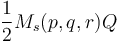$\frac{1}{2} M_s(p,q,r) Q$

- The second order model :

-- Integrates accelerate to obtain "stab_att_ref_rate" by the Euler method

-- Integrates rate to obtain "stab_att_ref_quat" by the Euler method

-- The Euler method uses dt. Here dt is implicite and equal 1/512 or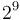$2^9$,

-- Determines the stab_att_ref_accel(12) by a second order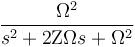$\frac{\Omega^2}{s^2+2 \Zeta \Omega s + \Omega^2}$ with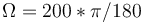$\Omega = 200*\pi/180$ and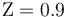$\Zeta = 0.9$.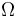$\Omega$ and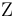$\Zeta$ are defined in airframe.xml.

• The second order
• Feedforward part
• Feedback part

With the assumption of small variations so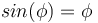$sin(\phi) = \phi$ and so on for pitch and yaw, terms of second order are ignored :
-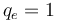$q_e = 1$
-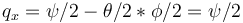$q_x = \psi/2 - \theta/2 * \phi/2 = \psi/2$
-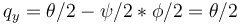$q_y = \theta/2 - \psi/2 *\phi/2 = \theta/2$
-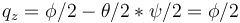$q_z = \phi/2 - \theta/2 * \psi/2 = \phi/2$